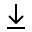MathBin Plane Member

A plane is a two-dimensional object that exists in two dimensions or higher. Specifically, it is a flat, two-dimensional surface. A plane is the two-dimensional analogue to a point (zero dimensions) and a line (one dimension).

In a Euclidean space of any number of dimensions, a plane is uniquely determined by any of the following:

• Three non-collinear points (points not on a single line).
• A line and a point not on that line.
• Two distinct but intersecting lines.
• Two parallel lines.

The following statements hold in three-dimensional Euclidean space but not in higher dimensions, though they have higher-dimensional analogues:

• Two distinct planes are either parallel or they intersect in a line.
• A line is either parallel to a plane, intersects it at a single point, or is contained in the plane.
• Two distinct lines perpendicular to the same plane must be parallel to each other.
• Two distinct planes perpendicular to the same line must be parallel to each other.

To find a plane given by three points involves finding the normal vector to the plane using those three points. There is no plane if the points are collinear (they are all on the same line).

Bins in Three Dimensions